# Introduction to Python

## Introduction

We must study python before we continue with machine learning because we will be using Python to apply the Machine Learning algorithms learned during the series.

This chapter provides you with an introduction to the Python language. During your voyage through the chapter, you will be going from Python Tokens to Python Classes.

## What is Python?

Python is an interpreted, high-level, general-purpose programming language. Created by Guido van Rossum and first released in 1991. he started python as a hobby project to keep him occupied in the week around Christmas. Got its name from the name of British comedy troupe Monty Python. It is used in :
1. Software Development
2. Web Development
3. System Scripting
4. Mathematics

## Installing Python

2. At the time of writing this, the latest version was 3.8.3.
For a more detailed guide on installing Python, visit

## Python Character Set

The following are then character set recognized by python. It uses the traditional ASCII character set.
1. Letters
A-Z, a-z

2. Digits
0-9

3. Special Symbols
Special Symbols available over Keyboard (some of the symbols like rupee symbol may not be available)

4. White Spaces
blank space, tab, carriage return, newline, form feed

5. Other Characters
Unicode

## Python Tokens

The smallest individual unit in a program is known as a token.
1. Keywords
There are in total of 31 reserved keywords. Ex: and, finally, not, etc.

2. Identifiers
a python identifier is a name used to identify a variable, function, class, module, or other objects. Ex: MyBook, myBook, mybook, etc.

3. Literals
Literals in Python can be defined as numbers, text, or other data that represent values to be stored in variables. Ex: age = 22, escape sequence, etc.

4. Operators
It can be defined as symbols that are used to perform operations on operands. Ex: arithmetic operators, etc.

5. Punctuators
Used to implement the grammatical and structure of a Syntax. Ex: &=, >>=, <<=, etc.

## Python Keywords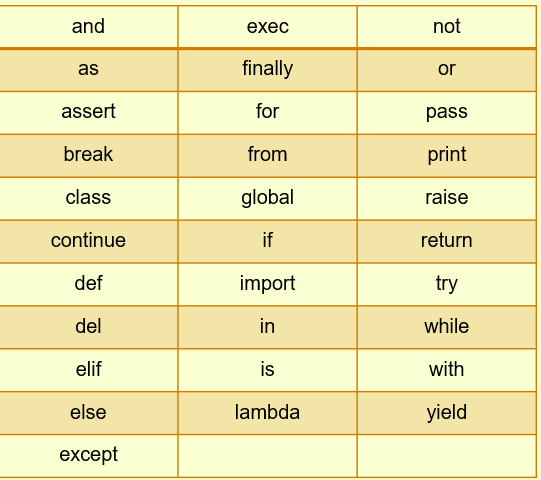## Rules to define Python Identifiers

1. An identifier starts with a letter A to Z or a to z or an underscore (_) followed by zero or more letters, underscores, and digits (0 to 9).
2. Python does not allow special characters
3. An identifier must not be a keyword of Python.
4. Python is a case sensitive programming language.
6. Starting an identifier with a single leading underscore indicates that the identifier is private.
7. Starting an identifier with two leading underscores indicates a strongly private identifier.
8. If the identifier also ends with two trailing underscores, the identifier is a language-defined special name.

## Python Punctuators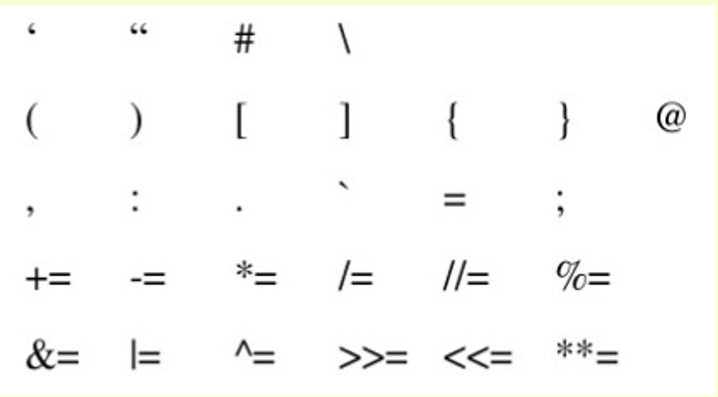## Barebone of a Python Program

1. Expression

Which is evaluated and hence produces results. Ex: (20=4)/4

2. Statements

Instructs that we are doing something.

Ex: a=20

print("Calling in proper sequence")

Which is readable for a programmer but ignored by the python interpreter.

a. Single line comment (#)

b. Multi-line comment (''' ''')

Ex: #this is a single-line comment

''' this is

multiple '''

4. Function

a code that has some name and it can be reused. Ex: KetArgFunc()

5. Block & Indentation

A group of statements in block indentation at the same level creates a block. Ex: all the statements that are under the function KetArgFunc()

## Python Variables

A variable can be considered as a container that holds value. Python is a type-infer language i.e. one which can detect data types automatically or in other words it means you dont need to specify the data type of variable.

Note: although python supports unlimited variable length, following PEP-8, we keep the maximum length to 79 characters

For example:
1. name = 'python' # String Data Type
2. sum =None # a variable without a value
3. a = 23 # integer
4. b = 6.2 # Float
5. sum = a+b
6. print(sum)

#### Multiple Assignments

1. a =b =c =1 #single value to multiple variables
2. a,b = 1, 2 #multiple value to multiple variable
3. a,b = b,a #value of a and b is swapped

1. Local Variable
1. def fun():
2.    x=8
3. print(x)
4.
5. fun()
6. print(x) #error will be shown
2. Global Variable
1. x = 8
2. def fun():
3.    print(x) #Calling variable 'x' inside fun()
4.
5. fun()
6. print(x) #Calling variable 'x' outside fun()

## Python Data Types

1. Number
It is used to store numeric values. Python has 3 numeric types:

a. Integers
Ex: a = 10

b. Floating Point numbers
Ex: a = 10.0

c. Complex numbers
Ex: a = complex(10,5) # 10+5j

2. String
A string is a sequence of characters. In python, we can create a string using a single ( ' ' ) or double quotes (" "). Both are same in python
1. str = 'computer science'
2. print('str- ', str) # print string
3. print('str-', str) # print 1st char
4. print('str[1:3]-', str[1:3]) # print string from position 1 to 3
5. print('str[3:]-', str[3:]) # print string starting from 3rd char
6. print('str*2-' , str*2) # print string two times
7. print('str + yes-', str+'yes') # concatenated string
3. Boolean
It is used to store two possible values either true or false
1. str = "comp sc"
2. b = str.isupper()
3. print(b)
4. List
List are collections of items and each item has its index value. Denoted by []
1. list = [6,9]
2. list = 55
3. print(list)
4. print(list)
5. Tuple
A tuple is an immutable Python Object. Immutable python objects mean you cannot modify the contents of a tuple once it is assigned. Denoted by ()
1. tup = (66,99)
2. Tup = 3 #error will be displayed
3. print(tup)
4. print(tup)
6. Set
It is an unordered collection of unique and immutable items. Denoted by {}
1. set1 = {11, 22, 33, 22}
2. print(set1)
7. Dictionary
It is an unordered collection of items and each item consists of a key and a value.
1. dict = {'subject' : 'comp sc", 'class' : '11'}
2. print(dict)
3. print( "Subject :", dict["subject"])
4. print("class :", dict.get('class'))

## Python Operators

The Precedence of Operators in Python can be found from the rule PEMDAS ( Parenthesis, Exponential, Multiply, Division, Addition, Subtraction). Operators with the same precedence are evaluated from left to right.
1. Python Arithmetic Operator

It is used for mathematical operations
1. x = 5
2. y = 4
3. print('x + y =',x+y) #Addition
4. print('x - y =',x-y) #Substraction
5. print('x * y =',x*y) #Multiplication
6. print('x / y =',x/y) #Division
7. print('x // y =',x//y) #Floor Division
8. print('x ** y =',x**y) #Exponent
2. Python Comparison Operators

used to compare values
1. x = 101
2. y = 121
3. print('x > y is',x>y) #Greater than
4. print('x < y is',x<y) #Less than
5. print('x == y is',x==y) #Equal to
6. print('x != y is',x!=y) #Not Equal to
7. print('x >= y is',x>=y) #Greater than equal to
8. print('x <= y is',x<=y) #Less than equal to
3. Python Logical Operators
1. x = True
2. y = False
3. print('x and y is',x and y) #if both are true
4. print('x or y is',x or y) #if either one is true
5. print('not x is',not x) #returns the complement
4. Python Bitwise Operators

Used to manipulate bit values
1. a = 6
2. b = 3
3. print ('a=',a,':',bin(a),'b=',b,':',bin(b)) c = 0
4. c = a & b
5. print ("result of AND is ", c,':',bin(c))
6. c = a | b
7. print ("result of OR is ", c,':',bin(c))
8. c = a ^ b
9. print ("result of EXOR is ", c,':',bin(c))
10. c = ~a
11. print ("result of COMPLEMENT is ", c,':',bin(c))
12. c = a << 2
13. print ("result of LEFT SHIFT is ", c,':',bin(c))
14. c = a>>2
15. print ("result of RIGHT SHIFT is ", c,':',bin(c))
5. Python Membership Operators

Test for membership in a sequence
1. a = 5
2. b = 10
3. list = [1, 2, 3, 4, 5 ]
4. if ( a in list ):
5.     print ("Line 1 - a is available in the given list")
6. else:
7.     print ("Line 1 - a is not available in the given list")
8. if ( b not in list ):
9.     print ("Line 2 - b is not available in the given list")
10. else:
11.     print ("Line 2 - b is available in the given list")
6. Python Identity Operators

They check if values on either side of equality operator point to the same object
1. a = 10
2. b = 10
3. print ('Line 1','a=',a,':',id(a), 'b=',b,':',id(b))
4. if ( a is b ):
5.    print ("Line 2 - a and b have same identity")
6. else:
7.    print ("Line 2 - a and b do not have same identity")

In computer programming, operator overloading, sometimes termed operator ad hoc polymorphism, is a specific case of polymorphism, where different operators have different implementations depending on their arguments. Operator overloading is generally defined by a programming language, a programmer, or both.

 Unary Operator Method - __neg__(self) + __pos__(self)

## Python Control Statements

Control statements are used to control the flow of execution depending upon the specified condition/logic.
1. If- Statement

An if statement is a programming conditional statement that, if proved true, performs a function or displays information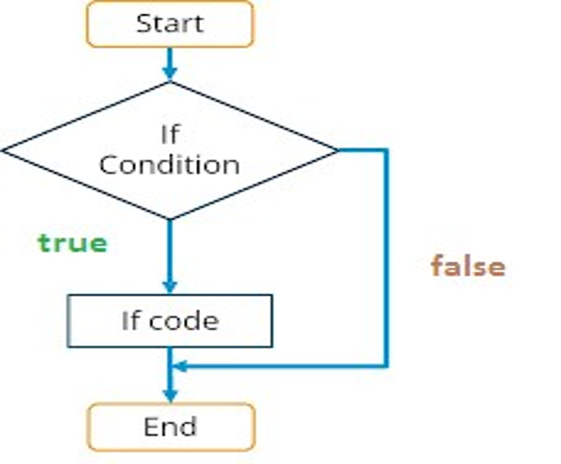1. noofbooks = 2
2. if (noofbooks == 2) :
3.    print(" You have")
4.    print("two books")
5. print("outside of if statement")
In the above code, we are checking if noofbooks is equal to 2, then execute the given code.

2. if-else statement

the if-else statement executes some code if the test expression is true (non-zero) and some other code if the test expression is false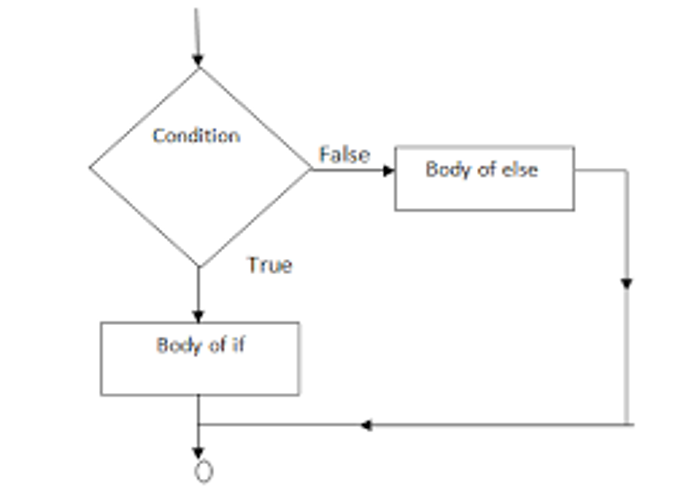1. a=10
2. if a<100:
3.       print('less than 100')
4. else:
5.       print('more than equal 100')
In the above code, we are checking if a is less than 100, else will print "more than equal 100'

3. Nested If-Else Statement

The nested if ... else statement allows you to check for multiple test expressions and executes different codes for more than two conditions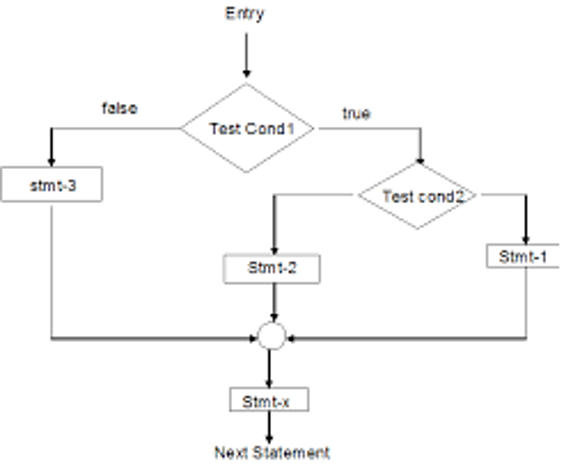1. num = float(input("enter a number:"))
2. if num >=0:
3.      if num==0:
4.          print("zero")
5. else:
6.      print("Negative number")
In the above code, we are first checking if num is greater than or equal to 0 and then if it is zero if both the conditions are met, "zero" is printed, otherwise "Negative number"

## Python Control Loops

1. While Loop

It is used to execute a block of a statement as long as a given condition is true. And when the condition becomes false, the control will come out of the loop. The condition is checked every time at the beginning of the loop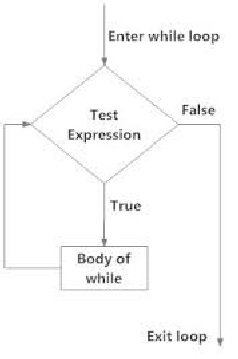1. x=1
2. while x<=4 :
3.       print(x)
4.       x = x+1
In the above code, we are printing all the values from 1 to 4

2. For Loop

It is used to iterate over items of any sequence, such as a list or a string.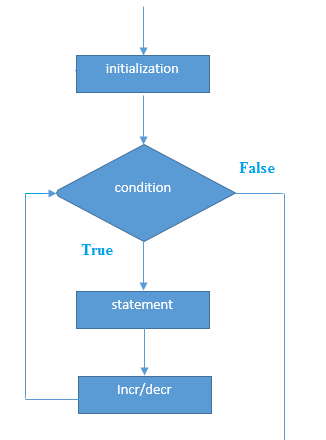1. for i in range (3,5):
2.       print(i)
3. In the above code, we are printing numbers from 3 to 5.

4. Nested For Loop

For loop within for loop is what we mean by nested for loops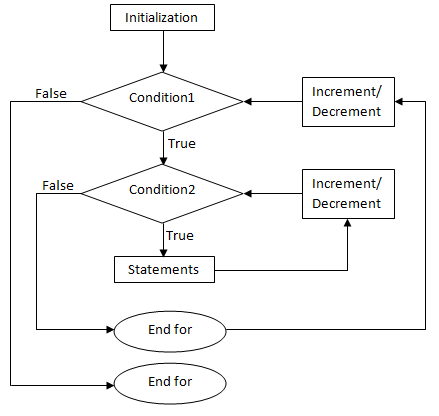1. for i in range (1,3):
2.     for j in range(1,11):
3.           k=i*j
4.           print(k, end = ' ')
5.     print()
5. In the above code, we are running our loop firstly from 1 to 3 and then for each iteration running for another 10 times.

## Python Jump Statements

They are used to transfer the program's control from one location to another. Means these are used to alter the flow of a loop like-to skip a part of a loop or terminate a loop.
1. Break

It is used to terminate the loop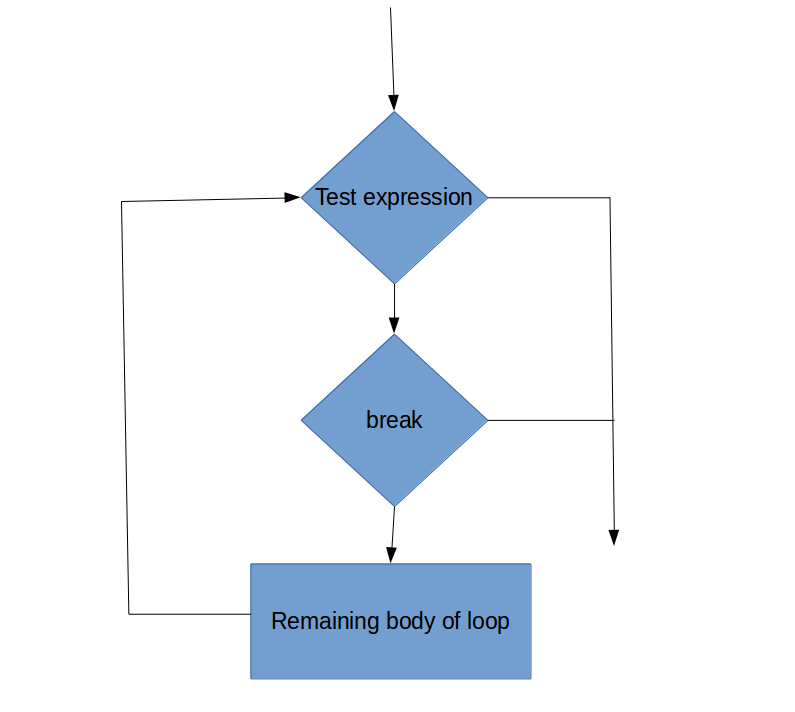1. for val in "string":
2.       if val == "i":
3.              break
4.       print(val)
2. In the above code, we break the loop as soon as "i" is encountered, hence printing "str"

3. continue

It is used to skip all the remaining statements in the loop and move controls back to the top of the loop.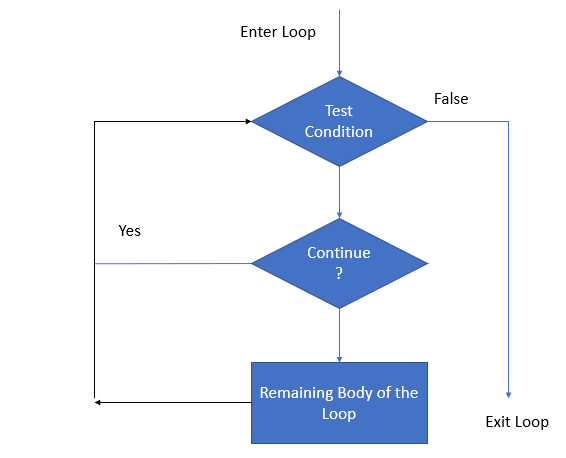1. for val in "init":
2.       if val == "i":
3.             continue
4.        print(val)
5. print(" The End"
4. In the above article, we will be printing "nt", because for "i" it will skip.

5. pass

This statement does nothing. It can be used when a statement is required syntactically but the program requires no action.
1. for i in "initial":
2.       if(i == "i"):
3.             pass
4.       else:
5.            print(i)
6. Continue forces the loop to start the next iteration while pass means "there is no code to execute here" and will continue through the remainder of the loop body.The output will be "ntal"

## Python Errors & Exception

### Errors

A Syntax error is an error in the syntax of a sequence of characters or tokens that are intended to be written in a particular programming language.

For Example:

>>> while True print 'Hello world'

In the above code, it is an Invalid Syntax error. It should be as :
>>> while True:
print 'Hello world'

Standard Errors available in Python are:
IndentationError, SystemExit, ValueError, TypeError, RuntimeError

### Exceptions

The other kind of error in Python are exceptions. Even if a statement or expression is syntactically correct, it may cause an error when an attempt is made to execute it. Errors detected during execution are called exceptions

For Example:
>> 10 * (1/10)

The above code is syntactically okay, but when we execute it, it will result in ZeroDivisionError: integer division or modulo by zero.

Standard Exceptions available in Python are:
SystenExit, OverflowError, FloatingPointError, ZeroDivisonError, EOFError, KeyboardInterrupt, IndexError, IOError

### Handling an Exception

If we see some suspicious code that may raise an exception, we can defend our program by placing the code in the try block

Syntax

try:
except Exception1:
if there is Exception1, then execute this block
except Exception2:
if there is Exception2, then execute this block
.........
else:
if there is no exception, then execute this block.
1. try:
2.    fh = open("testfile""r")
3.    fh.write("This is my test file for exception handling!!")
4. except IOError:
5.    print ("Error: can\'t find file or read data")
6. else:
7.    print ("Written content in the file successfully")

## Python List

 Function Description list.append() Add an item at end of a list list.extend() Add multiple items at the end of a list list.insert() insert an item at a defined index list.remove() remove an item from a lsit del list[index] delete an item from a list list.clear() empty all the list list.pop() remove an item at a defined index list.index() return index of the first matched item list.sort() sort the items of a list in ascending or descending order list.reverse() reverse the items of a list len(list) return total length of the list max(list) return item with maximum value in the list min(list) return item with the minimum value in the list list(seq) converts a tuple, string, set, dictionary into a list

## Python Dictionary

 Function Description dict.clear() removes all elements of dictionary dict dict.copy() returns a shallow copy of dictionary dict dict.items() retuns a list of dict's (key, value) tuple pairs dict.setdeafult(key, default=Nonre) similiar to get(), but will set dict[key] = default dict.update(dict2) adds dictionary dict2's key-values pairs to dict dict.keys() returns list of dictionary dict's keys dict.values() returns list of dictionary dict's values

## Python Tuples

 Function Description tuple(seq) converts a list into a tuple min(tuple) returns item from the tuple with a minimum value max(tuple) returns item from the tuple with maximum value len(tuple) gives the total length of the tuple cmp(tuple1,tuple2) compares elements of both tuples

## Python Class

A class is a code template for creating objects. Objects have member variables and have behavior associated with them. In python, a class is created by the keyword `class`. An object is created using the constructor of the class. This object will then be called the `instance` of a class. In Python, we create instances in the following manner.

#### Declaring a Class:

class name:
statements

#### Using a Class

1. class Point:
2.         x = 0
3.         y = 0
4.
5. # main
6. p1 = Point()
7. p1.x = 2
8. p1.y = -5
1. from Point import *
2.
3. # main
4. p1 = Point()
5. p1.x = 7
6. p1.y = -3
7. # Python objects are dynamic (can add fields any time!)
8. p1.name = "Tyler Durden"

#### Objects Methods

def name (self, parameter, ....., parameter):
statements

In the above code, self is very important, as, without the presence of self, python will not allow us to use any of the class member functions. It is similar to "this" used in Java. With only one difference that in java using "this" is not compulsory
1. from math import *
2.
3. class Point:
4.     x = 0
5.     y = 0
6.
7.     def set_location(self, x, y):
8.         self.x = x
9.         self.y = y
10.
11.     def distance_from_origin(self):
12.         return sqrt(self.x * self.x + self.y * self.y)
13.
14.     def distance(self, other):
15.         dx = self.x - other.x
16.         dy = self.y - other.y
17.         return sqrt(dx * dx + dy * dy)
In the above code, we have created 3 methods namely set_location(), distance_from_origin() and distance().

### Calling Methods

A client can call the methods of an object in two ways:
1. object.method( parameter)
2. Class.method( object, parameters)
1. p = Point(3, -4)
2. p.translate (15)
3. Point.translate (p, 15)

### Python Class Constructors

a constructor is a special method with the name ` __init__`

def __init__ (self, parameter, ....., parameter):
statements
1. class Point:
2.        def __init__( self, x, y):
3.                self.x = y
4.                self.y = y

### toString and __str__

It is equivalent to Java's `toString` which converts objects to a string. It is automatically involved when `str` or `print` is called.

def __str__( self):
return string
1. from math import *
2.
3. class Point:
4.     def __init__(self, x, y):
5.         self.x = x
6.         self.y = y
7.
8.     def distance_from_origin(self):
9.         return sqrt(self.x * self.x + self.y * self.y)
10.
11.     def distance(self, other):
12.         dx = self.x - other.x
13.         dy = self.y - other.y
14.         return sqrt(dx * dx + dy * dy)
15.
16.     def translate(self, dx, dy):
17.         self.x += dx
18.         self.y += dy
19.
20.     def __str__(self):
21.         return "(" + str(self.x) + ", " + str(self.y) + ")"